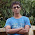### IQ Measurement Puzzle - Statistics Problem

Source: 40 Puzzles and Problems in Probability and Mathematical Statistics (Interesting book by Wolfgang Schwarz)

Inspiration: This problem demonstrates clearly the shortcomings of out grading system through exams

Problem:
Peter has an IQ of 90 whereas the IQ of Paula is 110. However, due to unsystematic biological or psychological day-to-day variation that is unrelated to the IQ per se, any single measurement of either IQ is distorted by an independent additive measurement error that has a zero-mean normal distribution with some variance. For example, if Paula’s IQ were measured repeatedly, the outcomes would be normally distributed with a mean of 110 (her “true” IQ) and some standard deviation. Suppose that either Peter or Paula is selected at random (p = 0.5), and his/her IQ is measured. You do not know who was selected, but you are told that the result of this ﬁrst measurement is 105. Now the same person —whose identity is unknown to you — is measured a second time.

a) What is your prediction for the outcome of this second measurement if standard deviation = 3?
b) Answer the same question if standard deviation = 20?

Update (Sep 15, 2012):
Minor change in the question as suggested by Akshay Soni

Update (5th Feb 2013):
Solution posted by Akshay Soni (IITB Mech Senior Undergraduate) , AB and Nikhil Simha R (Amazon India SDE, CSE IITB 2012 Alumnus) in comments! Rephrased and improved formatting of the solution and posted by me in comments!

1.Calculating the probability that peter was selected given the measurement,
P(Pe|measurement=m)=P(m|Pe)*P(Pe)/total probab
P(Pe|measurement=m)= N1(m)/N1(m)+N2(m)
Let the above quantity be U
where, N1~(m1,sigma^2) & N2~(m2,sigma^2)
Second measurement given the first = m1*U + m2*(1-U)
Substituting values:
new measurement=
(110+ 90*exp(-100/sigma^2))/(1+exp(-100/sigma^2)

Now with given variance, i.e. sigma^2=3, sigma^2=20,
new measurement ~ 110 which is close to Paula's

1.Correct solution. Thanks

2.I tried an extremely crude method for solving this.
I'll do the case for std. deviation 20:-
Since probability function for any particular point in a normal distribution is zero, I assumed a range between 104.99 and 105.01 for calculating the probability based on mean and std. deviation.
Corresponding to Paula, the probability of IQ between 104.99 and 105.01 is 0.00039 while for Peter it is 0.0003. Now applying Bayes rule, the probability of the score belonging to Paula is 0.5652 while for Peter it is 0.4348, hence the expected value of next IQ test will be 101.302.

1.Correct solution. Our answer do not match exactly because of rounding error. Range does not matter. We could have used pdf only. There was no need of cdf. The area "dx" would have cancelled in both numerator and denominator if you would have solved it using variables and then taken "dx" to zero. Nevertheless, correct solution. Thanks

3.p(p=peter|x=105)
=(p(x=105|p=peter)*p(p=peter))/(p(x=105|p=peter)*p(p=peter)+p(x=105|p=paula)*p(p=paula))
(bayes rule)
= p(x=105|p=peter)/p(x=105|p=peter)+p(x=105|p=paula)
( because p(p=peter)=p(p=paula)=1/2 )

prediction=p(p=peter|x=105)*90+p(p=paula|x=105)*110
p(p=paula|x=105)=mu(110,dev)(105)/(mu(110,dev)(105)+mu(90,dev)(105)) // mu(mean,deviation) is gaussian deviation prediction
now lets try putting dev=20 or dev=3;

when x=3 we see almost that 105 has its contribution almost all from paula and none from peter for your prediction. Hence approximately 110. (you are very sure it is paula who scored 105)

but at standard deviation 20 you begin to see that there is significant contribution from peter to predict the next value. ( you are not really sure who is the one who scored 105)

I don't know if this is what you had in mind. But looks like with one exam(high std. dev.) you cannot really determine who the score belonged to specially even if it is closer to paula's mean.
So I think you never can really estimate mean scores of students with just one(very less number of) exam.

1.Perfect solution and explanation. Thanks

4.5.Rephrasing the solution posted by Nikhil Simha. Thanks a ton

Say first measurement is x
P(Peter|x=105)=P(x=105|Peter)*P(Peter)/(P(x=105|Peter)*P(Peter)+P(x=105|Paula)*P(Paula))

Since P(Peter)=P(Paula) = 1/2

P(Peter|x=105)=P(x=105|Peter)/(P(x=105|Peter)+P(x=105|Paula))

Prediction for second measurement = P(Peter|x=105)*90+P(Paula|x=105)*110

P(Paula|x=105)=mu(110,dev)(105)/(mu(110,dev)(105)+mu(90,dev)(105))
// mu(mean,deviation) is gaussian deviation prediction
Putting dev=20 or dev=3;

P(Paula|x=105) with dev=3 is 0.033/(0.033+0.000) ~ 1
P(Paula|x=105) with dev=20 is 0.019/(0.019+0.015) = 0.559

Prediction with dev=3 is 110
Prediction with dev=20 is 90*(1-0.559)+110*(0.559) = 90+20*0.559 = 101.18

Discussion:
"When x=3 we see almost that 105 has its contribution almost all from Paula and none from Peter for your prediction. Hence approximately 110. (you are very sure it is Paula who scored 105)

but at standard deviation 20 you begin to see that there is significant contribution from Peter to predict the next value. ( you are not really sure who is the one who scored 105)"